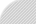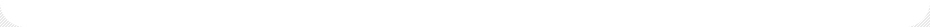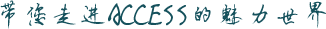VBA（高速版）转换为中文字日期-何保辛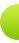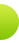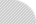Access软件网QQ交流学习群(群号码198465573),欢迎您的加入！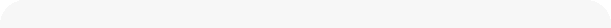VBA（高速版）转换为中文字日期 发表时间：2013/1/5 5:37:16　评论(0)　浏览(6808)　　评论 | 加入收藏 | 复制 摘　要:进入云计算年代了，将会是每一行代码算成本的年代，你的代码越精简、越高速，就能赢取更大的使用人群！ 正　文:

```Public Function CDateTime(MyDate As Date) As String
'大写中文日期
'引用:CDateTime(# 1968-1-24 #)
Dim i As Long, d(3) As String
For i = 1 To Len(Year(MyDate))
d(0) = d(0) & Mid("零一二三四五六七八九", CInt(Mid(Year(MyDate), i, 1)) + 1, 1)
Next
d(1) = "年" & Choose(Month(MyDate) \ 10 + 1, "", "十") & Mid(" 一二三四五六七八九", Month(MyDate) Mod 10 + 1, 1) & "月"
d(2) = Choose(Day(MyDate) \ 10 + 1, "", "十", "二十", "三十") & Mid(" 一二三四五六七八九", Day(MyDate) Mod 10 + 1, 1) & "日"
CDateTime = Join(d, "")
CDateTime = Replace(CDateTime, " ", "")
End Function```

```? CDateTime( now())

```

`select CDateTime( [日期]) as C_DATE from A_Table`

```t=timer(): for j=1 to 10000: n=CDateTime(now()): next: ? timer()-t
.3847656 ```

```Public Function DigitChi(d, cc, cd)
dtext = Format(d, "yyyymmdd")
Dim b() As Byte
b = dtext
For i = 0 To 15 Step 2: b(i) = b(i) - 48: Next
DigitChi = cc(b(0)) & cc(b(2)) & cc(b(4)) & cc(b(6)) & "年" & _
cd(b(8)) & cc(b(10)) & "月" & cd(b(12)) & cc(b(14)) & "日"
End Function```

cc, cd 则在呼叫前定义好

```    cc = Array("零", "一", "二", "三", "四", "五", "六", "七", "八", "九")
cd = Array("", "十", "二十", "三十")```

1. 用 Byte Array 来 处理文本，下面两句就能把文本放在一个 数组里面了
Dim b() as Byte
b = dtext
2. 由于Windows的Unicode是UCS-2BE的，所以，数字的 ascii 码会在第一个字节，然后第二个字节就为0。
输出的 b(0) , b(2), b(4),... b(14) 就是那8个数字的 ascii码。ascii 码减去 0的ascii码 48 就是 我们要 0-9的值。直接从 cc 与 cd 里面提取就行了！
3. 另外也是最关键的，cc 和 cd 其实是常数，VBA又不能定义 常数数组，唯有，所以在呼叫这个函数之前定义好，在运行的时候就不用在代码里解释这段字符串代码，毕竟VBA的编译器没办法固化这些数组！
（应用到窗体或者报表，这两个变量，最好 Public 代码预先生成，而就不需要传入参数了！）

```cc = Array("零", "一", "二", "三", "四", "五", "六", "七", "八", "九")
cd = Array("", "十", "二十", "三十")
t=timer(): for j=1 to 10000: n=DigitChi(now(),cc,cd): next: ? timer()-t
.1542969
```开发者你们好，这是 地球信息思维开发者 dbaseIIIer （QQ325613888)  更多的 Access/VBA 互联网交互技术，可以参考 本站内部栏目  互联网技术栏目 更多的思维在  新浪微博   Access贴吧  VisualPHP贴吧

Access软件网交流QQ群(群号：198465573)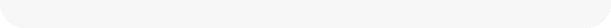相关文章如何把数字日期转成支票中文日期  【狸猫  2007/11/29】自定义中文日期显示函数  【十段  2008/6/21】窗体和报表中显示中文日期示例  【十段  2008/7/13】对＂窗体和报表中显示中文日期示例＂的改进  【符鸿敏  2008/7/14】短小精干_中文日期大写  【纵云梯  2012/2/13】

访客评论发表评论评论内容 (必填) 名字(必填)： 密码(必填)：
 常见问答
 技术分类
 相关资源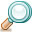文章搜索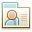关于作者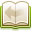文章分类文章存档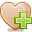友情链接